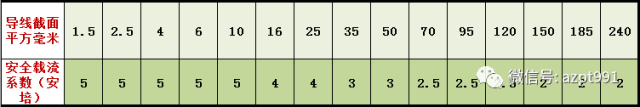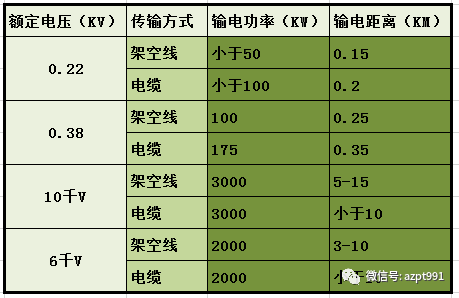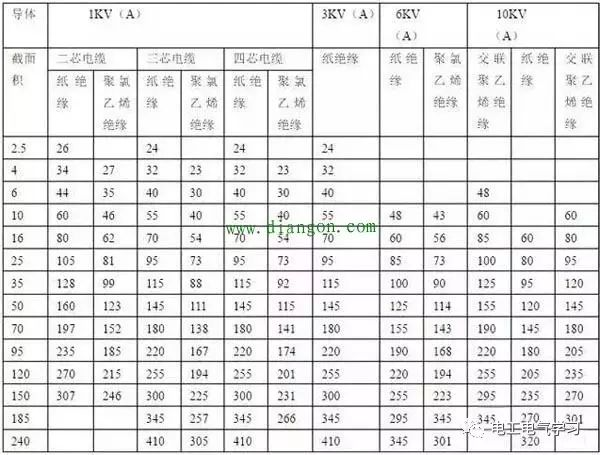# 最全面的电气设计估算公式！

2021/10/08

30

1、照明电路电流计算及熔丝刀闸的选择

(取系数1.1)
QS--------刀闸
IR---------熔丝

2 、380V/220V常用负荷计算

3、配电电力变压器的选择

=600/0.722=830千VA

=490/0.855=576千VA

=525／0.63=833千VA

4、功率因数的计算

=1000/1044=0.957

cosΦ=有功/=1000/
=1/1.22=0.81

5、电动机接触器热元件选择

（2）已知 U=380V P=10千瓦 cosΦ=0.85 n=0.95

=10/(1.73×0.38×0.85×0.95)=20(安)

6、绝缘导线的安全电流计算7、 380V三相电动机导线截面选择计算

8、低压380V/220V三相四线架空导线截面选择计算

S=P×系数×M
=30×4×0.2
=24平方毫米

S——导线截面
M——员荷距（千瓦/公里）

S=4×40×0.35
S=56平方毫米，整定为70平方毫米

S=24×20×0.1
S=48平方毫米，整定为50平方毫米1、铜芯电缆的载流量为表中数值乘以系数1.3倍；
2、本表格载流量为单根电缆容量；
3、单芯塑料电缆为三角形排列，中心距等于电缆外径。

1、铜芯电缆的载流量为表中数值乘以系数1.3倍；
2、本表格载流量为单根电缆容量；
3、单芯塑料电缆为三角形排列，中心距等于电缆外径。

</sec